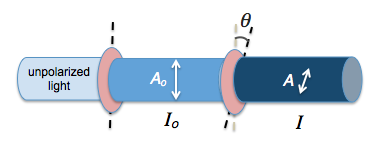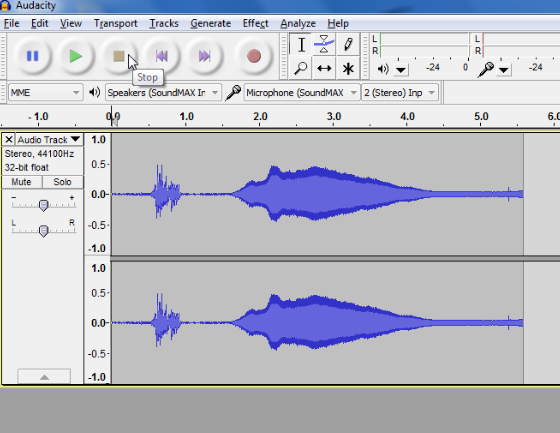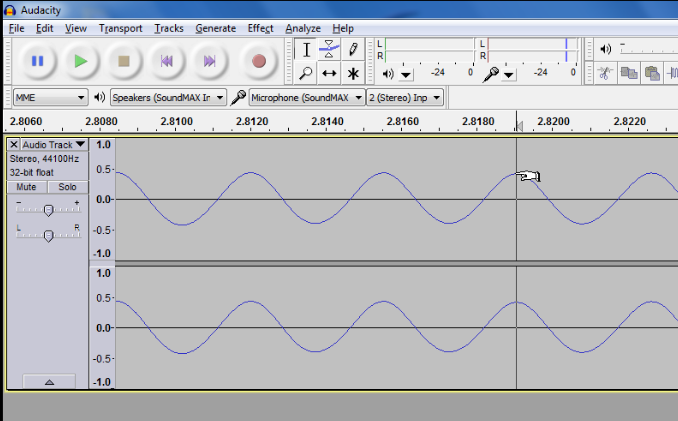# Month: May 2013

## Polarization with 3 Filters

In what seems like a counter-intuitive demonstration, we can place a polarizing filter in between two other filters which do not transmit light in order to cause light to pass through again.

This is because each filter will permit the components of electric field vectors of the electromagnetic waves that are parallel to its axis of polarization according to the equation $$A = A_o \cos{\alpha}$$ where $$A_o$$ is the original amplitude of the unpolarized wave incident on the filter and $$\alpha$$ is the angle between the electric field vector and the axis of polarization. Each time the wave passes through a filter, it undergoes a reduction in amplitude according to the equation so that by the third filter, its resultant amplitude is
$$A = A_o \cos{\alpha_1} \cos{\alpha_2}cos{\alpha_3}$$
where $$alpha_i$$ is the angle between the axis of polarization of the ith filter and the electric field vector direction of the incident light on the ith filter.

According to Malus’ law, which applies to two filters with an angle of $$\theta$$ between their axes of polarization, the resulting intensity for light that passes through 3 filters is given by
$$I=I_o \cos^2{\theta_1}\cos^2{\theta_2}$$
where $$\theta_1$$ is the angle between the axes of the first and second filters and $$theta_2$$ is the angle between the axes of the second and third filters.

## Polarization Using Sunglasses and a Computer Screen

Using a pair of polarizing sunglasses, you can demonstrate the effects of polarization together with a computer screen which is also polarizing. When the axes of polarization of the two polarizing screens are rotated, the brightness alternates between bright and dark.

Light coming from a computer screen is usually polarized. In the video below, when polarized light passes through another polarizer, the intensity of the light is given by Malus’ law:

$$I = I_o cos ^2{\theta}$$

where $$\theta$$ is the angle between the two axes of polarization and $$I_o$$ is the original intensity of the unpolarized light.

Only the components of electric field vectors in electromagnetic radiation that are parallel to the axis of polarization of a polarizing filter will be permitted through. Those electric field components that are perpendicular to the polarization axis are blocked by the filter.Hence, the amplitude of a vector A that passes through is given by $$A = A_o \cos{\theta}$$. Since intensity is proportional to the square of amplitude ($$I \propto {A^2}$$), we have Malus’ law.

The purpose of having polarizing filters in sunglasses and computer screens is to cut out glare due to light from other sources.

## MinutePhysics as an Educational Resource

As a teacher in a junior college running a 2-year pre-university course, I am often on the lookout for videos that can help me explain some of the more difficult concepts in physics. It is hard to come across videos that communicate scientific concepts in a way that is both informative and engaging.

When I stumbled upon MinutePhysics, a Youtube channel that aims to present a physics concept in roughly one minute, I was delighted. I have been sharing videos from this channel with my colleagues and students since then. The benefit of these videos is that they complement our lectures and help students improve their understanding of specific concepts in a very short time.

Here are just some videos that are relevant to my country’s curriculum. Our students take the Singapore-Cambridge GCE ‘A’ level Exams. Our syllabus covers topics such as quantum physics (including concepts such as quantum tunneling), nuclear physics, lasers and semiconductors.

On how lasers work:

On quantum tunneling:

On nuclear fusion and quantum tunneling:

On $$E=mc^2$$

## Learning about Sound with Audacity

Audacity is a free audio recording and editing software downloadable from http://audacity.sourceforge.net. It is cross-platform meaning you can run it on PC or Mac.

Once you have the software installed, you can try out some of its simpler functions, such as recording and playing back. You can also generate tones of known frequencies, which will be useful for experiments such as using stationary waves to determine the speed of sound in air.

Frequency and pitch

The first activity serves as an introduction to the software and can be easily carried out. All you need is a computer with Audacity installed and a microphone connected to it. Tuning forks of different frequencies will be best for this activity because of the simple and pure waveforms generated. A regular wave pattern can also be recorded with the help of a musical instrument such as a guitar or by singing a note.

To make a sound with the tuning fork, strike it against something hard such as the heel of your hand. The two prongs of the fork, known as “tines,”  will then vibrate with a fixed frequency, thus generating a waveform with a displacement that is almost sinusoidal. Place this tuning fork next to the microphone and you should see a densely packed waveform like the following:Zoom into the peaks recorded by clicking on the peaks of interest and pressing Ctrl 1 for Windows or Command 1 for Mac. You should observe a repeated pattern in the waveform.By reading off the time difference on the horizontal axis between two peaks, you can measure the period of the wave. Using $$f=\frac{1}{T}$$ where f is the frequency of the wave, and T is the period, you can verify the frequency measured with the known value of the tuning fork’s frequency.

In the above graph, the period is 2.8190-2.8155 = 0.0035s. The frequency of the tuning fork used is given by $$f=\frac{1}{T}=\frac{1}{0.0035}= 286 \text{ Hz}$$ which is roughly that of a D note.